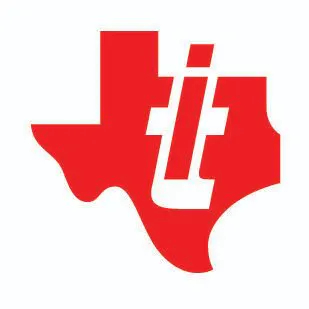Published

Cleaning Bot

A remote controlled and line following cleaning bot with a scrub attached on its back to clean the table and floor.

IntermediateFull instructions providedOver 1 day399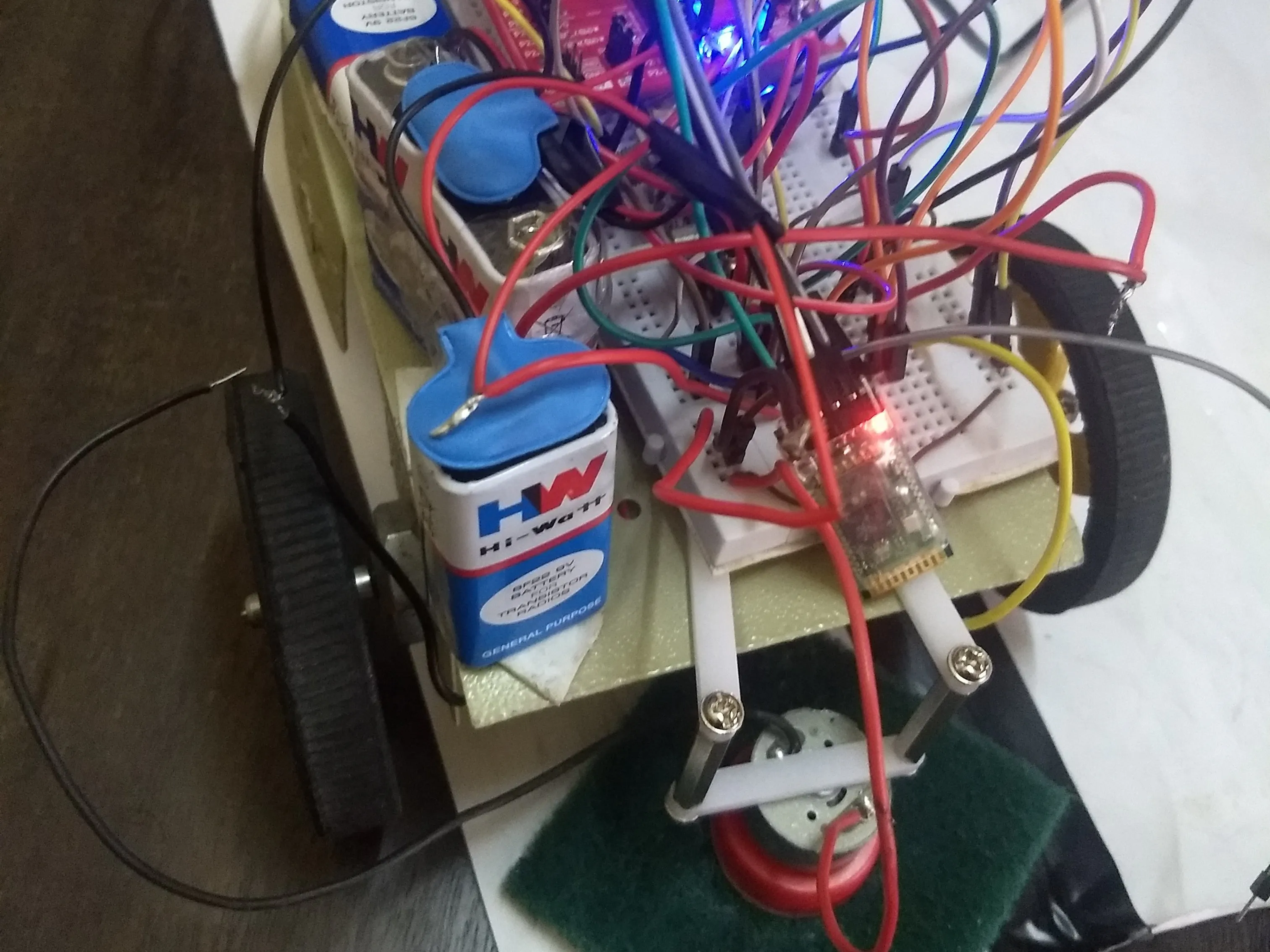Things used in this project

Hardware components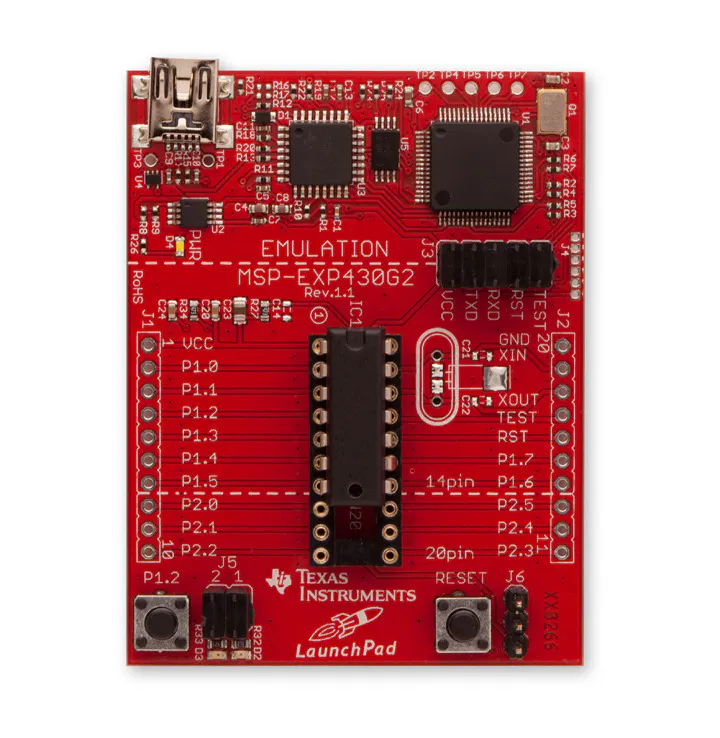Texas Instruments MSP-EXP430G2 MSP430 LaunchPad
×1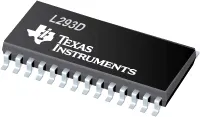Texas Instruments Dual H-Bridge motor drivers L293D
×1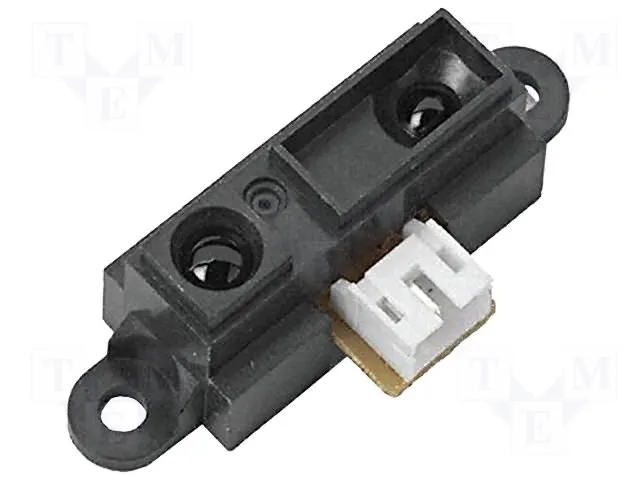Proximity Sensor
×1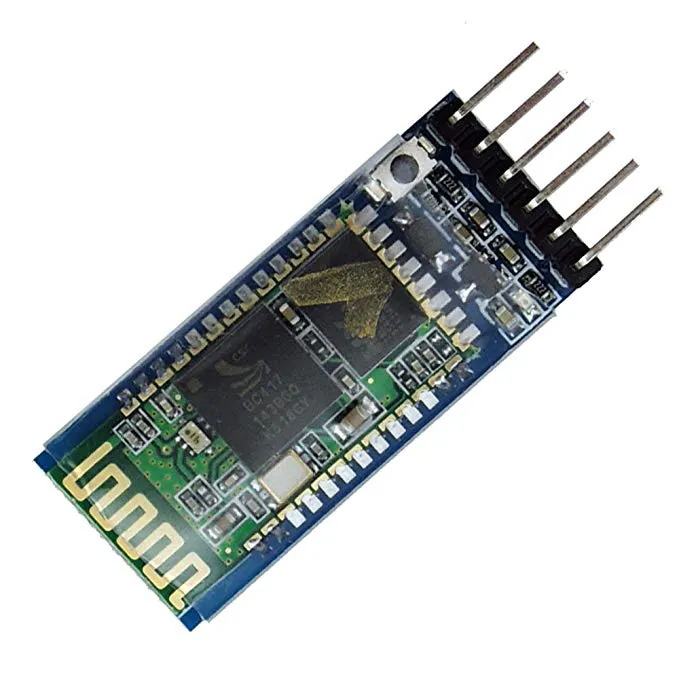HC-05 Bluetooth Module
×1Ultrasonic Sensor - HC-SR04 (Generic)
×1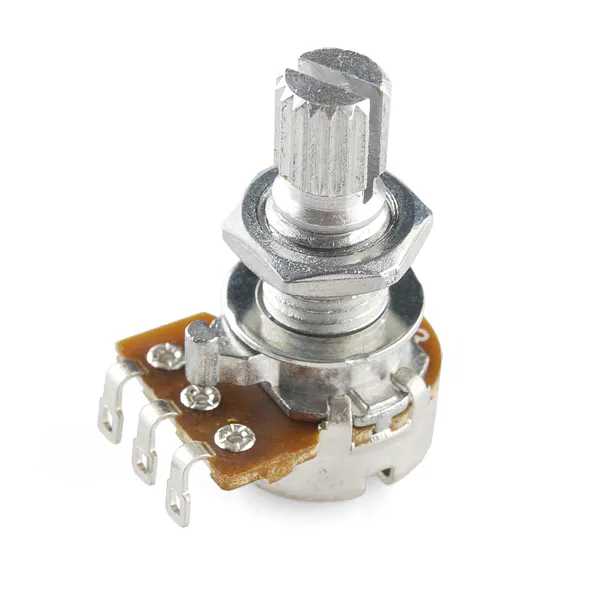Rotary potentiometer (generic)
×1

Software apps and online servicesTexas Instruments Energia

Code

cleaning boy

C/C++
#include <SoftwareSerial.h>

SoftwareSerial mySerial(P2_5, P2_7); // RX, TX

#define L_MTR_OUTPIN  P2_0  //PWM
#define l_minus P2_4
#define R_MTR_OUTPIN  P2_6
#define r_minus P2_3

#define L_IR_PIN P1_4
#define R_IR_PIN P1_5

#define DELL 30
#define MAXSPD 200
#define DARK_THRESH 100

int _lsp;
int _rsp;

// the setup routine runs once when you press reset:
void setup() {
// initialize serial communication at 9600 bits per second:
Serial.begin(9600); // msp430g2231 must use 4800

mySerial.begin(9600);

pinMode(L_MTR_OUTPIN, OUTPUT);
pinMode(R_MTR_OUTPIN, OUTPUT);
pinMode(r_minus,OUTPUT);
pinMode(l_minus,OUTPUT);

digitalWrite(l_minus, LOW);
digitalWrite(r_minus, LOW);

/* pinMode(TRG_PIN, OUTPUT);
pinMode(ECH_PIN, INPUT);  */

pinMode(L_IR_PIN, INPUT);
pinMode(R_IR_PIN, INPUT);

pinMode(P2_5,INPUT);
pinMode(P2_7,OUTPUT);

_lsp = _rsp = MAXSPD;

}

int _f = 0;
void loop()
{

{
_f = 0;

}

if(_f == 1)
{
{
digitalWrite(L_MTR_OUTPIN,HIGH);
digitalWrite(R_MTR_OUTPIN,HIGH);
//             digitalWrite(l_minus,LOW);
//               digitalWrite(r_minus,HIGH);
Serial.println("111111111111111");
delay(1000);

}
else if(dataReceived == '2'){
digitalWrite(L_MTR_OUTPIN,HIGH);
digitalWrite(R_MTR_OUTPIN,LOW);
digitalWrite(l_minus,LOW);
digitalWrite(r_minus,LOW);
Serial.println("222222222222222222");
delay(1000);
}else if(dataReceived == '3'){
digitalWrite(L_MTR_OUTPIN,HIGH);
digitalWrite(R_MTR_OUTPIN,HIGH);
//              digitalWrite(l_minus,LOW);
//              digitalWrite(r_minus,LOW);
Serial.println("33333333333333");
delay(1000);
}else if(dataReceived == '4'){
digitalWrite(L_MTR_OUTPIN,LOW);
digitalWrite(R_MTR_OUTPIN,HIGH);
//              digitalWrite(l_minus,HIGH);
//              digitalWrite(r_minus,HIGH);
Serial.println("4444444444444");
delay(1000);
}
}

if (_f == 0)
{
speedCheck();
run();
}
}

//checks the IR sensors to recalculate motor speeds
//caps between 0 and MAXSPD
void speedCheck()
{
int _leftir = analogRead(L_IR_PIN);
int _rightir = analogRead(R_IR_PIN);

//Left side
if (_leftir < DARK_THRESH)
_lsp -= DELL;
else
_lsp += DELL;

if (_lsp > MAXSPD)
_lsp = MAXSPD;
if (_lsp < 0)
_lsp = 0;

//Right side
if (_rightir < DARK_THRESH)
_rsp -= DELL;

else
_rsp += DELL;

if (_rsp > MAXSPD)
_rsp = MAXSPD;
if (_rsp < 0)
_rsp = 0;
}

//run both motors
void run()
{
analogWrite(L_MTR_OUTPIN, _lsp );
analogWrite(R_MTR_OUTPIN, _rsp );
}

Credits

prabhansh pandey

1 project • 2 followers

AbhiKalra

1 project • 2 followers

Texas Instruments University Program

91 projects • 84 followers
TI helps students discover what's possible to engineer their future. Learn about TI tech for the classroom: www.university.ti.com

Mohammed Ibrahim Ali

0 projects • 1 follower

Shashank Holla

0 projects • 1 follower